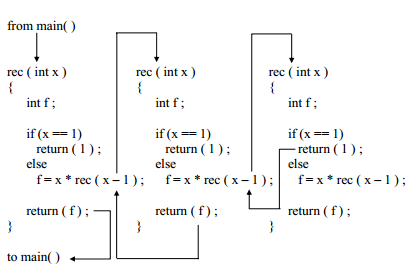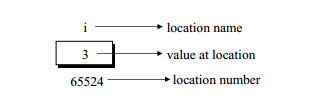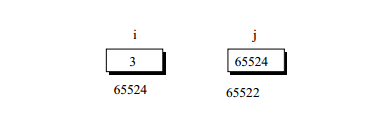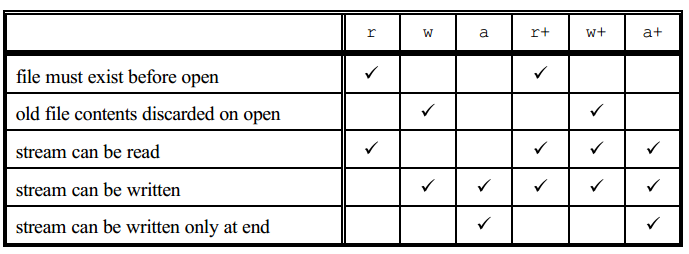## 1. C语言程序设计

### 1.1 什么是C语言？

C语言是1972年由Dennis Ritchie在美国 AT&T 公司贝尔实验室开发的一种编程语言。

• 面向问题的语言（高级语言）
• 面向机器的语言（低级语言）

### 1.2 C程序的特点

• 结构化语言
• 它具有划分和隐藏所有信息和指令的能力。
• C语言中的代码可以用函数或代码块来划分。
• 与其他语言相比，C语言是一种结构化的语言。
• 通用语言
• 使其成为系统编程的理想语言。
• 它也可以用于商业和科学应用。
• ANSI在1983年为c语言建立了一个标准。
• c语言拥有操纵位、字节和地址的能力。
• 它在1990年以后被采用。
• 可移植性
• 可移植性是指可以移植或使用编写的软件。
• 一个计算机C语言程序可以重复使用。
• 通过修改或不修改。
• 代码的可重用性&定制和扩展能力
• 一个程序员可以轻松地创建自己的函数。
• 它可以在不同的应用中反复使用。
• C程序基本上是函数的集合。
• 函数由'c'库支持。
• 函数可以持续添加到'c'库中。
• 关键字数量有限
• 在'C'中只有32个关键词。
• 里奇给出了27个关键词。
• 5个是由美国国家标准委员会添加的。
• 'C'的优势在于它的内置函数。
• Unix系统提供了尽可能多的C函数。
• 有些函数是在操作中使用。
• 其他的函数是用于专业的应用。

### 1.3 C程序结构

```pre-processor directives
global declarations
main()
{
local variable deceleration
statement sequences
function invoking
}
```

### 1.4 C关键词

```auto        double      int         struct
break       else        long        switch
case        enum        register    typedef
char        extern      return      union
const       float       short       unsigned
continue    for         signed      void
default     goto        sizeof      volatile
do          if          static      while
```

### 1.5 C字符集

• 字母 - A, B, ...., Y, Z a, b, ..., ..., y, z
• 数字 - 0、1、2、3、4、5、6、7、8、9
• 特殊符号 - ~ ' ! @ # % ^ & * ( ) _ - + = | { }[ ] : ; " ' < > , . ? /

### 1.6 C程序的编写、编译和执行规则

• C是区分大小写的，这意味着名为 "COUNTER "的变量与名为 "counter "的变量不同。
• 所有的关键字都是小写的。
• 关键词不能用于其他任何目的（如变量名）。
• 每个C语言的语句都必须以;结束。因此，;作为语句的终结者。
• 第一个字符必须是字母或下划线，除下划线外，不允许使用特殊符号，在变量、常量或关键字中不允许使用逗号或空格。
• 为了提高语句的可读性，可以在两个字之间插入空格。但是，在变量、常数或关键字中不允许有空格。
• 在程序中使用变量之前，必须先声明变量。
• 文件的扩展名应该是.c
• 程序在执行前必须先编译。

### 1.7 数据类型和占位符

• C语言有 5 种基本的内置数据类型。
• 数据类型定义了一个变量可以存储的一组值以及可以对其进行的一系列操作。
• 一个变量在不同的时间有不同的值。
• 声明一个变量的一般形式是:
```type name
```
• 下面是一个使用变量的例子。
```#include<stdio.h>
main()
{
int sum;
sum=12;
sum=sum+5;
printf("Sum is %d",sum);
}
```

printf 函数将打印以下内容:

Sum is 17

• int - integer
• char - character
• long - long integer
• float - float number
• double - long float

 Placeholders Format %c Character %d Signed decimal integer %i Signed decimal integer %e Scientific notation[e] %E Scientific notation[E] %f Decimal floating point %o unsigned octal %s String of character %u unsigned decimal integer %x unsigned Hexadecimal (lower) %X unsigned Hexadecimal (upper) %p dispaly a pointer %% print a %

### 1.8 控制字符（转义序列）

a - Bell n - New line r - Carriage return b - Backspace f - Formfeed（换页） t - 水平方向tab " - 引号 v - 竖直方向tab ' - Apostrophe \ - Backslash ? - 问号 0 - Null

### 1.9 从键盘接收输入值

scanf功能用于接收来自键盘的输入。 scanf函数的一般形式是

```scanf("Format string",&variable,&variable,...);
```

Format string 包含了我们要从键盘上接收的变量的占位符。在变量列表中的每一个变量名称前都有一个 & 号。 字符串是这个规则的例外，它们不会在前面加上这个符号。

```float a;
int n;
scanf("%d%f",&n,&a);
```

## 2. 表达式和运算符的优先级

```DESCRIPTION            OPERATORS    ASSOCIATIVITY
Function Expression ()      Left to Right
Array Expression    []      Left to Right
Structure Operator  ->      Left to Right
Structure Operator  .       Left to Right
Unary minus -       Right to Left
Increment/Decrement ++, --  Right to Left
One’s compliment    ~       Right to Left
Negation    !       Right to Left
Address of  &       Right to Left
Value of address    `*`     Right to Left
Type cast   (type)  Right to Left
Size in bytes       sizeof  Right to Left
Multiplication      `*`     Left to Right
Division    /       Left to Right
Modulus     %       Left to Right
Subtraction -       Left to Right
Left shift  <<      Left to Right
Right shift >>      Left to Right
Less than   <       Left to Right
Less than or equal to       <=      Left to Right
Greater than        >       Left to Right
Greater than or equal to    >=      Left to Right
Equal to    ==      Left to Right
Not equal to        !=      Left to Right
Bitwise AND &       Left to Right
Bitwise exclusive OR        ^       Left to Right
Bitwise inclusive OR        |       Left to Right
Logical AND &&      Left to Right
Logical OR  ||      Left to Right
Conditional ?:      Right to Left
Assignment  =, *=, /=, %=, +=, -=, &=, ^=, |=, <<=, >>=     Right to Left
Comma       ,       Right to Left
```

## 3. 决策控制结构

C有三个主要的决策指令--if语句、if-else 语句和 switch 语句。

### 3.1 if 语句

C使用关键字 if 来实现决策控制指令。if 语句的一般形式是这样的。

```//for single statement
if(condition)
statement;

//for multiple statement
if(condition)
{
block of statement;
}
```

```//for single statement
if(expression)
statement;

//for multiple statement
if(expression)
{
block of statement;
}
```

```if (3 + 2 % 5)
printf("This works");
```

### 3.2 if-else 语句

```if (expression)
{
block of statement;
}
else
statement;
```
• 在 if 之后的语句组被称为 if 块。同样，在 else 之后的语句组成了 else 块。
• else 只能在 if 块的下面。if 块中的语句和 else 块中的语句被缩进了右边。
• 如果 if 块中只有一条语句要执行，而 else 块中只有一条语句，我们可以把这对括号去掉。
• 和 if 语句一样，else 的默认范围也是紧接在 else 之后的语句。要覆盖这个默认范围，必须使用一对大括号。

### 3.3 嵌套多个 if-else

```if (expression1)
statement;
else
{
if (expression2)
statement;
else
{
block of statement;
}
}
```

### 3.4 if-else 阶梯/else-if 从句

else-if 是最一般的多向决策的写法。

```if(expression1)
statement;
else if(expression2)
statement;
else if(expression3)
statement;
else if(expression4)
{
block of statement;
}
else
statement;
```

### 3.5 switch 语句或控制语句

switch 语句是一种多向决策，它可以测试一个表达式是否匹配多个 常数整数值 中的一个，并相应地进行分支。 switch 让语句我们从众多的选择中做出决定，或者更正确的说法是switch-case-default， 因为这三个关键字共同构成了switch语句。

```switch (expression)
{
case constant-expression:
statement1;
statement2;
break;
case constant-expression:
statement;
break;
...
default:
statement;
}
```
• 在 switch...case 命令中，每个 case 就像一个简单的标签。一个标签决定了程序中的一个点，程序的执行必须从那里继续执行。switch语句将选择其中的一个 case 部分或 标签 来继续执行程序。程序将继续执行，直到到达break命令为止。
• break语句在 switch 结构中具有重要的作用。如果你删除了 break，程序的执行将继续到下一个 case 段，其余的所有 case 段都将被执行，直到switch块结束（而大多数时候，我们只希望一个case段被执行）。
• 如果没有一个 case 部分与 switch 匹配，那么 default 部分将被执行。

### 3.6 switch 与 if-else 阶梯比较

• 不能用 switch 来测试 float 表达式
• 不能有变量表达式（例如，case a+3 是错误的)
• 多个 case 不能使用相同的表达式

## 4. 循环控制结构

### 4.1 while loop

```//for single statement
while(expression)
statement;

//for multiple statement
while(expression)
{
block of statement
}
```

while的一般形式如下：

```initialise loop counter;
while (test loopcounter using a condition)
{
do this;
and this;
increment loopcounter;
}
```

### 4.2 for loop

for 循环类似于 while 循环，但它更复杂。for循环是由 决定循环运行次数 的 控制语句 和 命令 部分组成的。 命令部分可以是单个命令，也可以是一个命令块。

```//for single statement
for(control statement)
statement;

//for multiple statement
for(control statement)
{
block of statement
}
```

```for ( initialization; test condition; run every time command )
```
• initialization 部分只在 for 循环开始时执行一次。我们可以在这里初始化一个循环变量。
• test condition 是循环中最重要的部分。如果这个条件有效（true），循环将继续运行。如果条件无效（false），循环将终止。
• run every time command 部分将在每个循环周期中执行。我们通过这部分来达到终止循环的最终条件。例如，我们可以通过 增加 或 减少 循环变量的值的方式，在指定的循环次数后，让循环条件变得无效，for循环就可以终止。

### 4.3 do-while循环

while 和 for 循环在顶部测试终止条件。相比之下，C语言中的第三个循环，即 do-while，每次通过循环体后，在底部测试； 循环体总是至少执行一次。do-while 的语法是：

```do
{
statements;
}while (expression);
```

statements 被执行，然后对 expression 进行判断。如果为真，则再次执行 statements，以此类推。 当 expression 变为false时，循环终止。 经验表明，do-while 比 while 和 for 要少用得多。do-while 循环是为了保证循环内的语句至少执行一次。

### 4.4 break 和 continue 语句

```while (num<20)
{
printf("Enter score : ");
scanf("%d",&scores[num]);
if(scores[num]<0)
break;
}
```

Continue 语句可以在循环中使用。像 break 一样 continue 也可以改变程序的 flow。 但是它不会终止循环，只是跳过当前循环的剩余语句，返回循环的起始点。

```#include<stdio.h>
main()
{
while((ch=getchar())!='\n')
{
if(ch=='.')
continue;
putchar(ch);
}
}
```

### 4.5 Goto 和 标签

C语言提供了可以无限使用的 goto 语句，并提供了分支到的标签。goto 语句从来都不是必要的，在实际工作中， 如果没有 goto 语句，写代码几乎都很容易。我们在本书中没有使用 goto 语句。

```for ( ... )
{
for ( ... )
{
...
if (disaster)
{
goto error;
}
}
}
...
error:
/* clean up the mess */
```

## 5. 数组

### 5.1 数组的声明

```int marks ;
```

```int num = { 2, 4, 12, 5, 45, 5 } ;
int n[] = { 2, 4, 12, 5, 45, 5 } ;
float press[] = { 12.3, 34.2 -23.4, -11.3 } ;
```

### 5.2 访问数组中的元素

```int valueOfThirdElement = marks
```

### 5.3 将数据输入到数组中

```for(i = 0;i <= 29;i++)
{
printf("\nEnter marks");
scanf("%d", &marks[i]);
}
```

for 循环会使用户查询和接收学生的分数的过程重复30次。第一次通过循环时，i的值为0， 所以 scanf() 函数将导致输入的值被存储在数组元素 mark 中，即数组的第一个元素。 这个过程会重复进行，直到 i 变成 29。这是最后一次通过循环，这是一件好事，因为没有像mark这样的数组元素。

### 5.4 从数组中读取数据

```for ( i = 0 ; i <= 29 ; i++ )
sum = sum + marks[i] ;
avg = sum / 30 ;
printf ( "\nAverage marks = %d", avg ) ;
```

### 5.5 例子

```#include<stdio.h>
main()
{
int avg, i, sum=0;
int marks ; /*array declaration */
for ( i = 0 ; i <= 29 ; i++ )
{
printf ( "\nEnter marks " ) ;
scanf ( "%d", &marks[i] ) ; /* store data in array */
}
for ( i = 0 ; i <= 29 ; i++ )
sum = sum + marks[i] ; /* read data from an array*/
avg = sum / 30 ;
printf ( "\nAverage marks = %d", avg ) ;
}
```

## 6 字符串

### 6.1 什么是字符串？

```#include<stdio.h>
main()
{
char name;
scanf("%s",name);
printf("Hello, %s , how are you ?\n",name);
}
```

```Enter your name : Vineet
Hello, Vineet, how are you ?
```

```name='B';
name='r';
name='i';
name='a';
name='n';
name='\0';
```

### 6.2 注意点

• 字符串的长度不能超过字符数组的维数。这是因为C语言编译器不对字符数组进行边界检查。
• scanf()不能接收多字字符串。因此像 "Vineet Choudhary "这样的名字是不能接受的。

```#include<stdio.h>
main( )
{
char name ;
printf ("Enter your full name ") ;
gets (name) ;
puts ("Hello!") ;
puts (name) ;
}
```

```Enter your name Vineet Choudhary
Hello!
Vineet Choudhary
```

```char name ;
printf ("Enter your full name ") ;
scanf ("%[^\n]s", name) ;
```

### 6.3 标准库中的字符串函数

```strlen - 查找字符串的长度
strlwr - 将字符串转换为小写
strupr - 将字符串转换为大写
strcat - 在另一个字符串的末尾添加一个字符串
strncat - 将一个字符串的前 n 个字符添加到另一个字符串
strcpy - 将字符串复制到另一个字符串中
strncpy - 将一个字符串的前 n 个字符复制到另一个字符串中。
strcmp - 比较两个字符串
strncmp - 比较两个字符串中的前n个字符。
strcmpi - 对两个字符串进行比较，不考虑大小写（"i" 代表该函数忽略了case)
stricmp - 对两个字符串进行比较，不考虑大小写（与 strcmpi 一样)
strnicmp - 比较两个字符串的前n个字符，不考虑大小写
strdup - 复制一个字符串
strchr - 查找字符串中给定字符的首次出现的次数
strrchr - 查找字符串中给定字符的最后一次出现时间
strstr - 查找给定字符串在另一个字符串中的首次出现
strset - 将字符串的所有字符设置为给定字符
strnset - 将字符串中的前n个字符设置为给定字符
strrev - 反转字符串
```

## 7. 函数

### 7.1 什么是函数？

```#include<stdio.h>

/*Function prototypes*/
myfunc();

main()
{
myfunc();
}

/*Function Defination*/
myfunc()
{
printf("Hello, this is a test\n");
}
```

• 任何C语言程序都至少包含一个函数
• 如果一个程序只包含一个函数，那么它必须是 main()
• 如果一个 C 程序包含一个以上的函数，那么其中的一个而且只有一个必须是 main()，因为程序的执行总是以 main() 开始
• 一个C语言程序中的函数的数量没有限制
• 程序中的每个函数都是按照 main() 中的函数调用的顺序来调用的
• 在每个函数完成后，控制权返回到 main()。当 main() 中的函数调用用完后，程序结束。

### 7.2 为什么要使用函数

• 编写函数可以避免重复编写同样的代码
• 使用函数可以让写程序变得更容易，并且可以跟踪它们在做什么。

### 7.3 函数参数

```#include<stdio.h>
/* use function prototypes */
sayhello(int count);
main()
{
sayhello(4);
}

sayhello(int count)
{
int c;
for(c=0;c<count;c++)
printf("Hello\n");
}
```

### 7.4 函数的返回值

```int sum()
{
int a,b,c;
a=1;
b=4;
c=a+b。
reurn c;
}
```

```main()
{
.
.
.
return 0;
}
```

int 类型函数的默认返回值是 0，如果你没有在 main() 函数中插入返回值 0 或其他任何值， 则会自动返回一个 0 值。如果你打算在你的函数中返回一个 int 值，最好在你的函数头中提到返回值，并在函数中写上。

### 7.5 void 返回值

C语言中还有一个 void 类型的函数。void 类型的函数是不返回值的函数。你可以把不需要返回值的函数定义为 void 类型的函数。

```void test ()
{
/* fuction code comes here but no return value */
}
```

void 函数不能被赋值给一个变量，因为它不返回值。所以不能这样写：

```a=test();
```

### 7.6 递归函数

```#include<stdio.h>
int rec(int);
main()
{
int a, fact ;
printf("\nEnter any number ");
scanf ("%d", &a);
fact = rec(a);
printf ("Factorial value = %d", fact);
}
int rec (int x)
{
int f;
if (x == 1)
return (1);
else
f = x * rec (x - 1) ;
return (f) ;
}
```

```Enter any number 5
Factorial value = 120
```

```rec(5) returns(5 * rec(4),
which returns (4 * rec(3),
which returns (3 * rec(2),
which returns (2 * rec(1),
which returns (1)))))
```### 7.7 多参数

```#include<stdio.h>
int min(int a,int b);
main()
{
int m;
m=min(3,6);
printf("Minimum is %d",m);
return 0;
}
int min(int a,int b)
{
if(a<b)
return a;
else
return b;
}
```

### 7.8 按值调用

C 语言编程语言中的函数调用，使用按值调用方法。让我们看一个例子来更好地理解这个话题。

```#include<stdio.h>
void test(int a);
main()
{
int m;
m=2;
printf("\nM is %d",m);
test(m);
printf("\nM is %d\n",m);
return 0;
}
void test(int a)
{
a=5;
}
```

```M is 2
M is 2
```

### 7.9 通过引用调用

```#include<stdio.h>
void test(int *ptr);
main()
{
int m;
m=2;
printf("\nM is %d",m);
test(&m);
printf("\nM is %d\n",m);
return 0;
}
void test(int *ptr)
{
printf("\nModifying the value inside memory address%ld",&m);
*ptr=5;
}
```

```ptr=&m ;
```

```scanf("%d",&a) ;
```

```ptr=&a ;
```

```val=*ptr; /* finding the value ptr points to */
```

```*ptr=5 ;
```

M is 2 Modifying the value inside memory address 2293620 M is 5

## 8. 指针

### 8.2 C指针语法

```<variable_type> *<name> ;
```

```int *points_to_integer ;
```

### 8.3 指针符号

```int i = 3;
```

• 在内存中预留空间来保存整数值。
• 将名称 i 与这个内存位置关联起来。
• 将值 3 存储在这个位置。
• 我们可以用下面的内存图来表示 i 在内存中的位置。```#include<stdio.h>
main()
{
int i = 3 ;
printf("\nValue of i = %d",i);
}
```

```Address of i = 65524
Value of i = 3
```

C语言中的另一个指针操作符是 * 叫做 value at address 操作符。 它给出了存储在某个特定地址的值。 value at address 操作符也被称为 indirection 操作符。

```#include<stdio.h>
main()
{
int i = 3 ;
printf("\nValueof i = %d",i);
printf("\nValue of i = %d",*(&i));
}
```

```Address of i = 65524
Value of i = 3
Value of i = 3
```

```j = &i ;
``````int *j ;
```

```int *j ;
```

### 8.4 例子

```#include<stdio.h>
main( )
{
int i = 3 ;
int *j ;
j = &i ;
printf ("\nAddress of i = %u",&i) ;
printf ("\nAddress of i = %u",j) ;
printf ("\nAddress of j = %u",&j) ;
printf ("\nValue of j = %u",j) ;
printf ("\nValue of i = %d",i) ;
printf ("\nValue of i = %d",*(&i)) ;
printf ("\nValue of i = %d",*j) ;
}
```

```Address of i = 65524
Value of j = 65524
Value of i = 3
Value of i = 3
Value of i = 3
```

## 9. 结构

### 9.1 结构的声明

```struct <structure name>
{
structure element 1;
structure element 2;
structure element 3;
......
......
structure element n;
};
```

```struct book
{
char name;
float price;
int pages;
};
```

```struct book b1, b2, b3 ;
```

```struct book
{
char name;
float price;
int pages;
};

struct book b1 = { "Basic", 130.00, 550 } ;
struct book b2 = { "Physics", 150.80, 800 } ;
```

### 9.2 访问结构元素

```b1.pages
```

```b1.price
```

### 9.3 示例

```#include<stdio.h>
main()
{
struct book
{
char name;
float price;
int pages;
};
struct book b1, b2, b3 ;

printf("\nEnter names, prices & no. of pages of 3 books\n");
scanf("%c %f %d", &b1.name, &b1.price, &b1.pages);
scanf("%c %f %d", &b2.name, &b2.price, &b2.pages);
scanf("%c %f %d", &b3.name, &b3.price, &b3.pages);

printf("\n\nAnd this is what you entered");
printf("\n%c %f %d", b1.name, b1.price, b1.pages);
printf("\n%c %f %d", b2.name, b2.price, b2.pages);
printf("\n%c %f %d", b3.name, b3.price, b3.pages);
}
```

```Enter names, prices and no. of pages of 3 books
A 100.00 354
C 256.50 682
F 233.70 512

And this is what you entered
A 100.000000 354
C 256.500000 682
F 233.700000 512
```

### 9.4 总结

• 当我们希望将不同的数据存储在一起时，通常会使用结构
• 结构元素可以通过结构变量使用点(.)运算符访问结构元素
• 结构元素可以使用箭头(->)运算符通过指向结构的指针来访问结构元素
• 一个结构变量的所有元素都可以通过赋值(=)运算符分配给另一个结构变量
• 可以通过值或地址将结构变量传递给一个函数
• 可以创建一个结构数组

## 10. 文件

### 10.1 文件指针

```FILE *fp;
```

fp 是一个指向文件的指针。

FILE 类型不是基本类型，而是在头文件 stdio.h 中定义的，这个文件必须包含在你的程序中。

### 10.2 打开一个文件

```fp = fopen(filename, mode);
```

filename 和 mode 都是字符串。mode 可以是：

• r - 读
• w - 写入，若存在，则覆盖
• a - 写入，若存在，则附加
• r+ - 读和写，若存在，不覆盖
• w+ - 读和写，若存在，覆盖
• a+ - 读和写，若存在，附加
• b - 可以附加到上述任何一个中，以强制以二进制模式而不是文本模式打开文件
• fp = fopen("data.dat", "a"); - 将打开磁盘文件data.dat进行写入，任何写入的信息都将附加到文件中```#include <stdio.h>
int main()
{
char filename;
FILE *fp;
printf("File to be opened? ");
scanf("%79s", filename);
fp = fopen(filename,"r");
if (fp == NULL)
{
fprintf(stderr, "Unable to open file %s\n", filename);
return 1; /* Exit to operating system */
}
//code that accesses the contents of the file
return 0;
}
```

• fprintf(fp, formatstring , ...) - 打印到一个文件。
• fscanf(fp, formatstring , ...) - 从文件中读取
• getc(fp) - 从文件中获取一个字符
• putc(c, fp) - 将一个字符放到文件中
• ungetc(c, fp) - 将一个字符回推到文件上（只保证一个字符可以回推）
• fopen( filename , mode) - 打开一个文件
• fclose(fp) - 关闭一个文件

```fprintf(sterr, "ERROR: unable to open file %s\n", filename);
```

fscanf() 和 getc() 函数用于顺序访问文件，也就是说，后续的读取将在刚刚读取的数据之后读取数据， 最终会到达文件的终点。如果你想在文件中向前或向后移动，或者从开始、结束或当前位置跳转到特定的偏移量， 可以使用 fseek() 函数(尽管文件必须以二进制模式打开)。函数 ftell() 报告从文件的起始位置开始的当前偏移量。

## 11. 命令行参数

```int main (int argc, char *argv[])
```

```filecopy PR1.C PR2.C
```

• argv - 将包含字符串 "filecopy" 的基本地址。
• argv - 将包含字符串 "PR1.C" 的基本地址。
• argv - 将包含字符串 "PR2.C" 的基本地址。